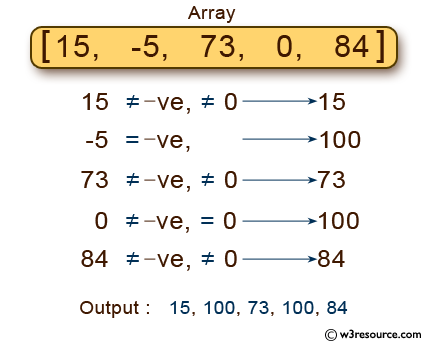﻿ C : Elements of an array of length 7 replacing some values

# C Exercises: Read and print the elements of an array of length 7 replacing some values

## C Basic Declarations and Expressions: Exercise-48 with Solution

Write a C program that reads and prints the elements of an array of length 7. Before printing, replace every negative number, zero, with 100.

Pictorial Presentation:Sample Solution:

C Code:

``````#include <stdio.h>
int main() {
int n, i, x;

// Input the 5 members of the array
printf("Input the 5 members of the array:\n");
for(i = 0; i < 5; i++) {
scanf("%d", &x);
if(x > 0) {
n[i] = x;
} else {
n[i] = 100;
}
}

// Print the array values
printf("Array values are: \n");
for(i = 0; i < 5; i++) {
printf("n[%d] = %d\n", i, n[i]);
}

return 0;
}
``````

Sample Output:

```Input the 5 members of the array:
25
45
35
65
15
Array values are:
n = 25
n = 45
n = 35
n = 65
n = 15
```

Flowchart:C programming Code Editor:

What is the difficulty level of this exercise?

Test your Programming skills with w3resource's quiz.

﻿